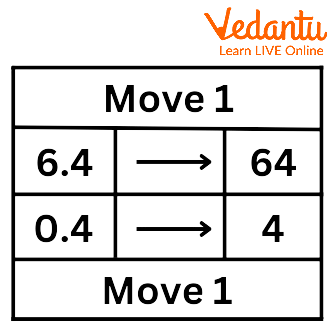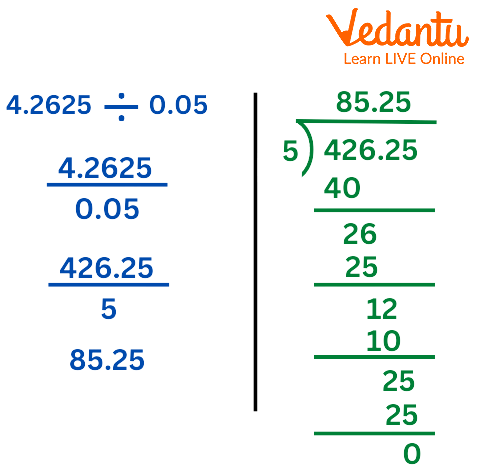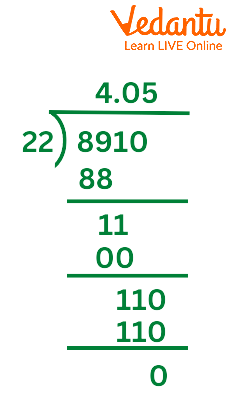Courses
Courses for Kids
Free study material
Free LIVE classes
More

# Division of Decimal by DecimalLIVE
Join Vedantu’s FREE Mastercalss

## Introduction to Decimals

Keeping the decimal point in mind, dividing decimals is similar to dividing entire numbers. Although there are specific guidelines to follow when splitting decimal numbers, the fundamental division procedure is the same. In this article, we will discuss long division with decimals, dividing decimals with whole numbers, and dividing decimals with other decimals.

## Division of Decimal by Decimal

When dividing decimals, we must move the decimal point to the right in order to change the divisor to a whole number. The decimal point of the dividend is then moved the same number of places to the right, and the numbers that result are divided in the same manner as conventional long division.

Let us move one space for both:

6.4/0.4 is exactly the same as 64/4 as we did the move for both numbers. Refer to the following diagram.How to Divide Decimals

Now we can calculate: 64/4=16

## How to Divide Decimal Numbers

To multiply one decimal by another:

The divisor's decimal point should be moved to the right until a whole number is reached.

To convert the divisor to a whole number, move the dividend's decimal point right by the same amount of spaces as the decimal point was moved.

Divide the new dividend by the new divisor after that.

The divisor should be multiplied by as many 10s as necessary to make it a whole number.

The dividend should be multiplied by the same amount of 10s.

Let’s understand it more clearly by dividing 4.2625 by 0.05.

Step 1: Write the number in fraction form like denominator and numerator.

Step 2: As we need to make the 0.05 as 5 we need more two decimals towards the left it will become then 5.00 which is 5. As we are changing the denominator we need to move two decimals from the numerator it will become from 4.2625 to 426.25

Step 3: Divide the 426.25 by 5.

Refer to the following Diagram.How to Divide Decimals

## Decimal Division by 10, 100, and 1000

Let's calculate the decimal division of a number by 10, 100, and 100

Think about ${\rm{97}}{\rm{.5 }} \div 10 = 9.75$. The decimal point has moved (to the left) in the quotient, but the digits in 97.5 and 9.75 are the same, i.e., 9, 7, and 5. As a result, when dividing a decimal by 10, the decimal point will move one place to the left because there is only one zero over one in 10.

From the previous illustration, we may understand that when decimals are divided by 10, 100, or 1000, the number and quotient digits will match. The decimal point in the quotient, however, is shifted to the left by an amount equal to the number of zeros above 1.

## Conclusion

The decimal point must be moved to the right when dividing decimals in order to convert the divisor to a whole number. The numbers that result are split using traditional long division after the decimal point of the dividend is moved the same number of places to the right.

## Decimal by Decimal Solved Examples

Example 1: Divide 8.91 by 2.2

By doing the long division method, we will get

The dividend is set at 8.91 and Divisor is 2.2

By moving the decimal point one place to the right, you may now convert the divisor 2.2 to a whole number. Next, move the decimal point one place to the right, keeping the same spacing, in the dividend.

$8.91 \div 2.2 = 8910 \div 22$Divide 8.91 by 2.2.

Example 2: How are decimals divided into steps?

Following is a step-by-step process for dividing decimals by whole numbers:

teSp 1: Write the division numbers in a sequence.

Step 2: The decimal number's whole number component must now be divided by the divisor.

Step 3: After placing the quotient's decimal point above the dividend's decimal point, bring the tenth digit down.

Step 4: Enter 0 in the quotient and in front of the tenth digit if the acquired dividend's tenth digit cannot be divided by the divisor. If not, continue with the division process.

Step 5: Carry on with the previous step, adding zeros to the left number until the remainder equals zero.

Example 3: How to divide any number by 10?

The decimal point should be one place to the left when dividing a decimal number by 10. For instance, we may easily divide 12.87 by 10 by moving the decimal point to the left, yielding the result of 1.287.

Last updated date: 24th Sep 2023
Total views: 66.9k
Views today: 2.66k

## FAQs on Division of Decimal by Decimal

1. When dividing by 10, which way do you shift the decimal point?

The decimal point advances one place to the left when a decimal is divided by 10.

2. How do you divide 5 by 100?

Only the decimal point needs to be in the numerator when dividing 5 by 100, as in$5 \div 100 = \dfrac{5}{{100}} = 0.05$. As a result of 100 having two 0s, the decimal point will move two positions to the left.

3. What decimal value is 31 over 100?

You may write 31 over 100 as $\dfrac{{31}}{{100}}$. The denominator in this case has two zeros. Therefore, the decimal point must come after two digits, as in 0.31.

4. How Do You Divide 100 Decimal Places?

If we divide a decimal number by 100, the decimal point shifts two positions to the left.

5. A decimal can be a whole number?

No, it cannot be a whole number because any number to the right of a decimal point has a value lower than 1.